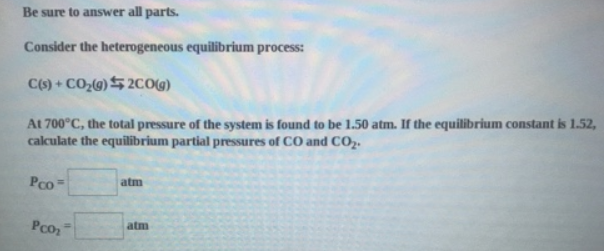# Problem: Be sure to answer all parts. Consider the heterogeneous equilibrium process: C(s) + CO2(g) ⇋ 2CO(g) At 700°C, the total pressure of the system is found to be 1.50 atm. If the equilibrium constant is 1.52, calculate the equilibrium partial pressures of CO and CO2.

###### FREE Expert Solution
95% (428 ratings)###### Problem Details

Be sure to answer all parts.

Consider the heterogeneous equilibrium process:

C(s) + CO2(g) ⇋ 2CO(g)

At 700°C, the total pressure of the system is found to be 1.50 atm. If the equilibrium constant is 1.52, calculate the equilibrium partial pressures of CO and CO2.